﻿

###$\ast$-Ricci soliton on GSSF with Sasakian metric

#### Abstract

We study generalized Sasakian-space-forms (GSSF)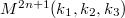$M^{2n+1} (k_1, k_2, k_3)$ with Sasa\-kian metric admitting$\ast$-Ricci soliton. We obtain that either such GSSF has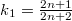$k_1=\frac{2n+1}{2n+2}$,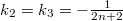$k_2= k_3=-\frac{1}{2n+2}$ and$\ast$-soliton is steady or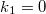$k_1=0$,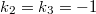$k_2=k_3=-1$ and$\ast$-soliton is expanding. Also, we provide some examples in support of results. Further, we give an example that GSSF with Sasakian metric with$k_1 \neq 0$ and$k_1 \neq \frac{2n+1}{2n+2}$ do not admit the$\ast$-Ricci soliton.

DOI Code: 10.1285/i15900932v42n1p95

Keywords: *-Ricci soliton; Generalized Sasakian-space-forms; Sasakian manifolds; Positive-Sasakian; Null-Sasakian

Full Text: PDF